Custom SearchPRACTICE PROBLEMS: Find the answers to the following: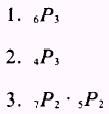a. A and B must be boys and C and D must be girls? b. two of the officers must be boys and two of the officers must be girls? ANSWERS: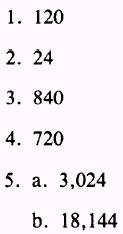If we were asked how many different arrangements of the letters in the word STOP can be made, we would writeWe would be correct since all letters are different. If some of the letters were the same, we would reason as given in the following problem. EXAMPLE: How many different arrangements of the letters in the word ROOM can be made? SOLUTION: We have two letters alike. If we list the possible arrangements, using subscripts to make a distinction between the O's, we have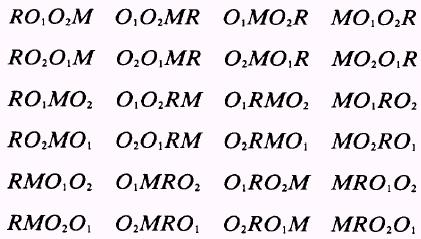but we cannot distinguish between the O's; RO1O2M and RO2O1M would be the same arrangement without the subscript. Only half as many arrangements are possible without the use of subscripts (a total of 12 arrangements). This leads to the statement: The number of arrangements of n items, where there are k groups of like items of size r1,r2, . . . rk, respectively, is given by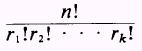in the previous example n was equal to 4 and two letters were alike; therefore, we would write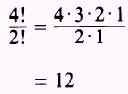EXAMPLE: How many arrangements can be made using the letters in the word ADAPTATION? SOLUTION: We usewhere n=10 and r1 = 2 (two T's) and r2 = 3 (three A's) Then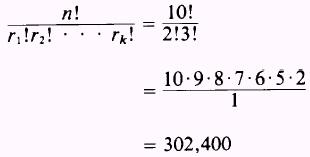PRACTICE PROBLEMS: Find the number of possible arrangements of the letters in the following words: 1. WRITE 2. STRUCTURE 3. HERE 4. MILLIAMPERE 5. TENNESSEE ANSWERS: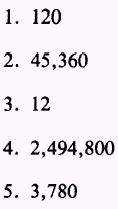Although the previous discussions have been associated with formulas, problems dealing with combinations and permutations may be analyzed and solved in a more meaningful way without complete reliance upon the formulas. EXAMPLE: How many four-digit numbers can be formed from the digits 2, 3, 4, 5, 6, and 7 a. without repetition? b. with repetition? SOLUTION: The (a) part of the question is a straightforward permutation problem, and we reason that we want the number of permutations of six items taken four at a time. Therefore,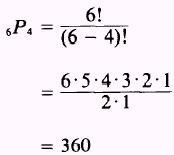The (b) part of the question would become quite complicated if we tried to use the formulas; therefore, we reason as follows: We desire a four-digit number and find we have six choices for the first position; that is, we may use any of the digits 2, 3, 4, 5, 6, or 7 in the first position: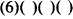Since we are allowed repetition of numbers, then we have six choices for the second position. In other words, any of the digits 2, 3, 4, 5, 6, or 7 can be used in the second position:Continuing this reasoning, we also have six choices each for the third and fourth positions: (6)(6)(6)(6) Therefore, the total number of four-digit numbers formed from the digits 2, 3, 4, 5, 6, and 7 with repetition isEXAMPLE: Suppose, in the previous example, we were to find how many three-digit odd numbers could be formed from the given digits without repetition. SOLUTION: We would be required to start in the units column because an odd number is determined by the units column digit. Therefore, we have only three choices for the units position; that is, either 3, 5, or 7: For the ten's position, we have only five choices, since we are not allowed repetition of numbers: Using the same reasoning of no repetition, we have only four choices for the hundred's position: (3)(5)(4) Therefore, we can form 3.5.4 = 60 three-digit odd numbers from the digits 2, 3, 4, 5, 6, and 7 without repetition.Integrated Publishing, Inc. - A (SDVOSB) Service Disabled Veteran Owned Small Business